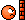# E=mc squared explanation

So, I've been viewing this calculator website, and from it i figured out how to do the Lorentz Contraction and Time Dilation formula. However, When i stumbled upon this one... No matter how i tried, I couldn't figure it out. So, Please help.

http://keisan.casio.com/exec/system/1224060366

jtbell
Mentor
What specifically is confusing you about it?

What specifically is confusing you about it?
Well.. I understand the square root part, because that is in all the formulas, but i don't exactly understand what the m0 means, and i have no clue as to how to get the E0. Oh, And sorry, I forgot to post the website. Posting now.

Mister T
Gold Member
##m_o## is the mass. ##E_o## is the rest energy. ##E_o=m_oc^2##.

##m_o## is the mass. ##E_o## is the rest energy. ##E_o=m_oc^2##.
I know all that, The problem is,I do the square root part... And then im not sure how to divide that. Because, I multiply the mass with speed of light, divide the result with the square root part, And then run it through the calculator to find its inconsistent with my results.

Last edited:
jtbell
Mentor
The problem is,I do the square root part...
What square root?

What square root?
The square root at the bottom part of the fraction.

jtbell
Mentor
What fraction? I don't see one in ##E_0 = m_0 c^2##. Isn't that the equation we're talking about?

Argh.I finally clicked your link. Sorry about that. Now I think I see what you're asking about.

How about showing us exactly what numbers you're trying to plug in, and the intermediate steps of your arithmetic? Then someone can probably tell you where you're going wrong.

What fraction? I don't see one in ##E_0 = m_0 c^2##. Isn't that the equation we're talking about?

Argh.I finally clicked your link. Sorry about that. Now I think I see what you're asking about.

How about showing us exactly what numbers you're trying to plug in, and the intermediate steps of your arithmetic? Then someone can probably tell you where you're going wrong.
Well, I know that when i do the square root, its right, because the calculator confirms. However, I then tried to divide m0 x c2, which worked. So then i declared that must be the value of E0, But then i get confused because i realize that the E0 is a fraction and that messes up my thought processes.

HallsofIvy
Homework Helper
The formula you link to says that
$$E=\frac{m_0c^2}{\sqrt{1-\frac{v^2}{c^2}}}$$

If you take, say, $m_0= 1 kg$ and $v= c/2= 299792.5/2=149896.25 km/s.$ then that formula says that
$$E= \frac{c^2}{\sqrt{1- \frac{1}{4}}}= \frac{c^2}{\frac{\sqrt{3}}{2}}= \frac{2c^2}{\sqrt{3}}$$ which would be 103779337954.2 Joules.

That is, much to my surprise, what I get when I enter the values into that website.

Janus
Staff Emeritus
Gold Member
$E_0 = m_0c^2$ is the "rest energy" of m0. It is the energy equivalence of the rest mass m0

$E = \frac{E_o}{\sqrt{1-\frac{v^2}{c^2}}} = \frac{m_oc^2}{\sqrt{1-\frac{v^2}{c^2}}}$ is the energy of m0 when moving at velocity v. (If v=0, then E=E0= m0c2)

Well.. I understand the square root part, because that is in all the formulas, but i don't exactly understand what the m0 means, and i have no clue as to how to get the E0. Oh, And sorry, I forgot to post the website. Posting now.
I don't know if anyone will be able to help until you post your calculations step by step.

Regardless, E0 is not going to include you dividing by the square root. It's just rest mass times the square of the speed of light. You don't even need the square root for rest energy. If you want to get the relativistic mass energy, then you divide by the square root.

Khashishi
That square root factor is common in relativity so there's a special name for it https://en.wikipedia.org/wiki/Lorentz_factor:
$$\gamma = \frac{1}{\sqrt{1-\frac{v^2}{c^2}}}$$
so
$$E = \gamma m c^2$$
It just means that an object moving at a faster velocity has more energy than an object at rest. How much more? Well that's what the formula tells you. The kinetic energy T is equal to the total energy minus the rest energy.
$$T = E - E_0 = (\gamma-1) m c^2$$
Note that for small ##v##, ##\gamma-1 \approx \frac{1}{2} \frac{v^2}{c^2}## (why don't you verify this with some trial numbers, or Taylor expansion if you know how?) so ##T \approx \frac{1}{2} m v^2##

•vanhees71
Khashishi
$$E^2 = m^2 c^4 + p^2 c^2$$
•